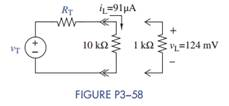### Create an Account

Home / Questions / You need to determine the Thévenin equivalent circuit of a more complex linear circuit A t...

# You need to determine the Thévenin equivalent circuit of a more complex linear circuit A technician tells you she made two measurements using her DMM The first was with a 10-kΩ load and the load

You need to determine the Thévenin equivalent circuit of a more complex linear circuit. A technician tells you she made two measurements using her DMM. The first was with a 10-kΩ load and the load current was 91 μA. The second was with a 1-kΩ load and the load voltage was 124 mV. Calculate the Thévenin equivalent circuit as shown in Figure P3−58.Jun 15 2020 View more View LessSubscribe To Get Solution